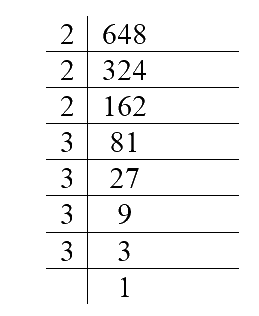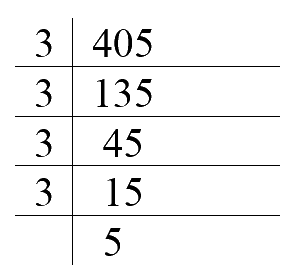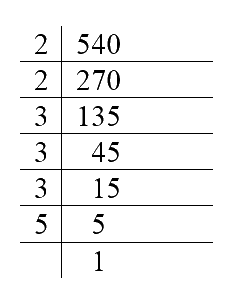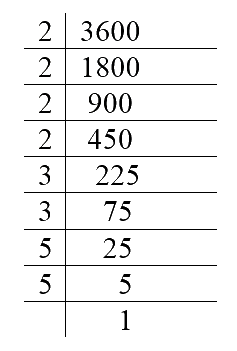# Ex 13.1 Q5 Exponents and Powers Solution- NCERT Maths Class 7

Go back to  'Ex.13.1'

## Question

Express each of the following as product of powers of their prime factors:

(i) $$648$$

(ii) $$405$$

(iii) $$540$$

(iv) $$3,600$$

Video Solution
Exponents And Powers
Ex 13.1 | Question 5

## Text Solution

What is known?

Numbers.

What is unknown?

Prime factors and powers of given numbers.

Reasoning:

In this question, first find prime factors of the given number and then raise the prime factor to its powers.

Steps:

(i) $$648$$Prime factorization of $$648$$ $$= 2 \times 2 \times 2 \times 3 \times 3 \times 3 \times 3$$

In this, $$3$$ is the exponent of $$2$$ and $$4$$ is the exponent of $$3$$

So, $$648$$ can be expressed as product of powers of their prime factors as $$2^3 \times$$$$3^4$$

(ii) $$405$$Prime factorization of $$405$$ $$= 3 \times3 \times 3 \times 3 \times 5$$

In this, $$4$$ is the exponent of $$3$$ and $$1$$ is the exponent of $$5$$

So, product of powers of prime factors $$=$$ $$3^3 \times$$ $$5$$

(iii) $$540$$Prime factorization of $$540$$ $$= 2 \times 2 \times 3 \times 3 \times 3 \times 5$$

In this, $$2$$ is the exponent of $$2$$. $$3$$ is the exponent of $$3$$ and $$1$$ is the exponent of $$5$$

So, product of powers of prime factors $$= 2^2 \times 3^3 \times 5$$

(iv) $$3,600$$Prime factorization of $$540$$ $$= \left[ \begin{array}{l}2 \times 2 \times 2 \times 2 \times \\3 \times 3 \times 5 \times 5\end{array} \right]$$

In this, $$4$$ is the exponent of $$2$$. $$2$$ is the exponent of $$3$$ and $$2$$ is the exponent of $$5$$

So, product of powers of prime factors $$= 2^4 \times 3^2 \times 5^2$$

Learn from the best math teachers and top your exams

• Live one on one classroom and doubt clearing
• Practice worksheets in and after class for conceptual clarity
• Personalized curriculum to keep up with school